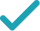Type a math problemSolve for xSteps Using Factoring
Steps Using Factoring By Grouping
Steps for Completing the Square
Steps Using Direct Factoring Method
Steps Using Factoring
To solve the equation, factor using formula . To find and , set up a system to be solved.
Since is negative, and have the opposite signs. Since is positive, the positive number has greater absolute value than the negative. List all such integer pairs that give product .
Calculate the sum for each pair.
The solution is the pair that gives sum .
Rewrite factored expression using the obtained values.
To find equation solutions, solve and .
GraphGraph Both Sides in 2D
Graph in 2DGiving is as easy as 1, 2, 3
Get 1,000 points to donate to a school of your choice when you join Give With Bing
a+b=1 ab=-56
To solve the equation, factor x^{2}+x-56 using formula x^{2}+\left(a+b\right)x+ab=\left(x+a\right)\left(x+b\right). To find a and b, set up a system to be solved.
-1,56 -2,28 -4,14 -7,8
Since ab is negative, a and b have the opposite signs. Since a+b is positive, the positive number has greater absolute value than the negative. List all such integer pairs that give product -56.
-1+56=55 -2+28=26 -4+14=10 -7+8=1
Calculate the sum for each pair.
a=-7 b=8
The solution is the pair that gives sum 1.
\left(x-7\right)\left(x+8\right)
Rewrite factored expression \left(x+a\right)\left(x+b\right) using the obtained values.
x=7 x=-8
To find equation solutions, solve x-7=0 and x+8=0.
a+b=1 ab=1\left(-56\right)=-56
To solve the equation, factor the left hand side by grouping. First, left hand side needs to be rewritten as x^{2}+ax+bx-56. To find a and b, set up a system to be solved.
-1,56 -2,28 -4,14 -7,8
Since ab is negative, a and b have the opposite signs. Since a+b is positive, the positive number has greater absolute value than the negative. List all such integer pairs that give product -56.
-1+56=55 -2+28=26 -4+14=10 -7+8=1
Calculate the sum for each pair.
a=-7 b=8
The solution is the pair that gives sum 1.
\left(x^{2}-7x\right)+\left(8x-56\right)
Rewrite x^{2}+x-56 as \left(x^{2}-7x\right)+\left(8x-56\right).
x\left(x-7\right)+8\left(x-7\right)
Factor out x in the first and 8 in the second group.
\left(x-7\right)\left(x+8\right)
Factor out common term x-7 by using distributive property.
x=7 x=-8
To find equation solutions, solve x-7=0 and x+8=0.
x^{2}+x-56=0
All equations of the form ax^{2}+bx+c=0 can be solved using the quadratic formula: \frac{-b±\sqrt{b^{2}-4ac}}{2a}. The quadratic formula gives two solutions, one when ± is addition and one when it is subtraction.
x=\frac{-1±\sqrt{1^{2}-4\left(-56\right)}}{2}
This equation is in standard form: ax^{2}+bx+c=0. Substitute 1 for a, 1 for b, and -56 for c in the quadratic formula, \frac{-b±\sqrt{b^{2}-4ac}}{2a}.
x=\frac{-1±\sqrt{1-4\left(-56\right)}}{2}
Square 1.
x=\frac{-1±\sqrt{1+224}}{2}
Multiply -4 times -56.
x=\frac{-1±\sqrt{225}}{2}
x=\frac{-1±15}{2}
Take the square root of 225.
x=\frac{14}{2}
Now solve the equation x=\frac{-1±15}{2} when ± is plus. Add -1 to 15.
x=7
Divide 14 by 2.
x=\frac{-16}{2}
Now solve the equation x=\frac{-1±15}{2} when ± is minus. Subtract 15 from -1.
x=-8
Divide -16 by 2.
x=7 x=-8
The equation is now solved.
x^{2}+x-56=0
Quadratic equations such as this one can be solved by completing the square. In order to complete the square, the equation must first be in the form x^{2}+bx=c.
x^{2}+x-56-\left(-56\right)=-\left(-56\right)
Add 56 to both sides of the equation.
x^{2}+x=-\left(-56\right)
Subtracting -56 from itself leaves 0.
x^{2}+x=56
Subtract -56 from 0.
x^{2}+x+\left(\frac{1}{2}\right)^{2}=56+\left(\frac{1}{2}\right)^{2}
Divide 1, the coefficient of the x term, by 2 to get \frac{1}{2}=0.5. Then add the square of \frac{1}{2}=0.5 to both sides of the equation. This step makes the left hand side of the equation a perfect square.
x^{2}+x+\frac{1}{4}=56+\frac{1}{4}
Square \frac{1}{2}=0.5 by squaring both the numerator and the denominator of the fraction.
x^{2}+x+\frac{1}{4}=\frac{225}{4}
\left(x+\frac{1}{2}\right)^{2}=\frac{225}{4}
Factor x^{2}+x+\frac{1}{4}. In general, when x^{2}+bx+c is a perfect square, it can always be factored as \left(x+\frac{b}{2}\right)^{2}.
\sqrt{\left(x+\frac{1}{2}\right)^{2}}=\sqrt{\frac{225}{4}}
Take the square root of both sides of the equation.
x+\frac{1}{2}=\frac{15}{2} x+\frac{1}{2}=-\frac{15}{2}
Simplify.
x=7 x=-8
Subtract \frac{1}{2}=0.5 from both sides of the equation.
x ^ 2 +1x -56 = 0
Quadratic equations such as this one can be solved by a new direct factoring method that does not require guess work. To use the direct factoring method, the equation must be in the form x^2+Bx+C=0.
r + s = -1 rs = -56
Let r and s be the factors for the quadratic equation such that x^2+Bx+C=(x−r)(x−s) where sum of factors (r+s)=−B and the product of factors rs = C
r = -\frac{1}{2} - u s = -\frac{1}{2} + u
Two numbers r and s sum up to -1 exactly when the average of the two numbers is \frac{1}{2}*-1 = -\frac{1}{2}. You can also see that the midpoint of r and s corresponds to the axis of symmetry of the parabola represented by the quadratic equation y=x^2+Bx+C. The values of r and s are equidistant from the center by an unknown quantity u. Express r and s with respect to variable u. <div style='padding: 8px'><img src='https://opalmath.azureedge.net/customsolver/quadraticgraph.png' style='width: 100%;max-width: 700px' /></div>
(-\frac{1}{2} - u) (-\frac{1}{2} + u) = -56
To solve for unknown quantity u, substitute these in the product equation rs = -56
\frac{1}{4} - u^2 = -56
Simplify by expanding (a -b) (a + b) = a^2 – b^2
-u^2 = -56-\frac{1}{4} = -\frac{225}{4}
Simplify the expression by subtracting \frac{1}{4} on both sides
u^2 = \frac{225}{4} u = \pm\sqrt{\frac{225}{4}} = \pm \frac{15}{2}
Simplify the expression by multiplying -1 on both sides and take the square root to obtain the value of unknown variable u
r =-\frac{1}{2} - \frac{15}{2} = -8 s = -\frac{1}{2} + \frac{15}{2} = 7
The factors r and s are the solutions to the quadratic equation. Substitute the value of u to compute the r and s.